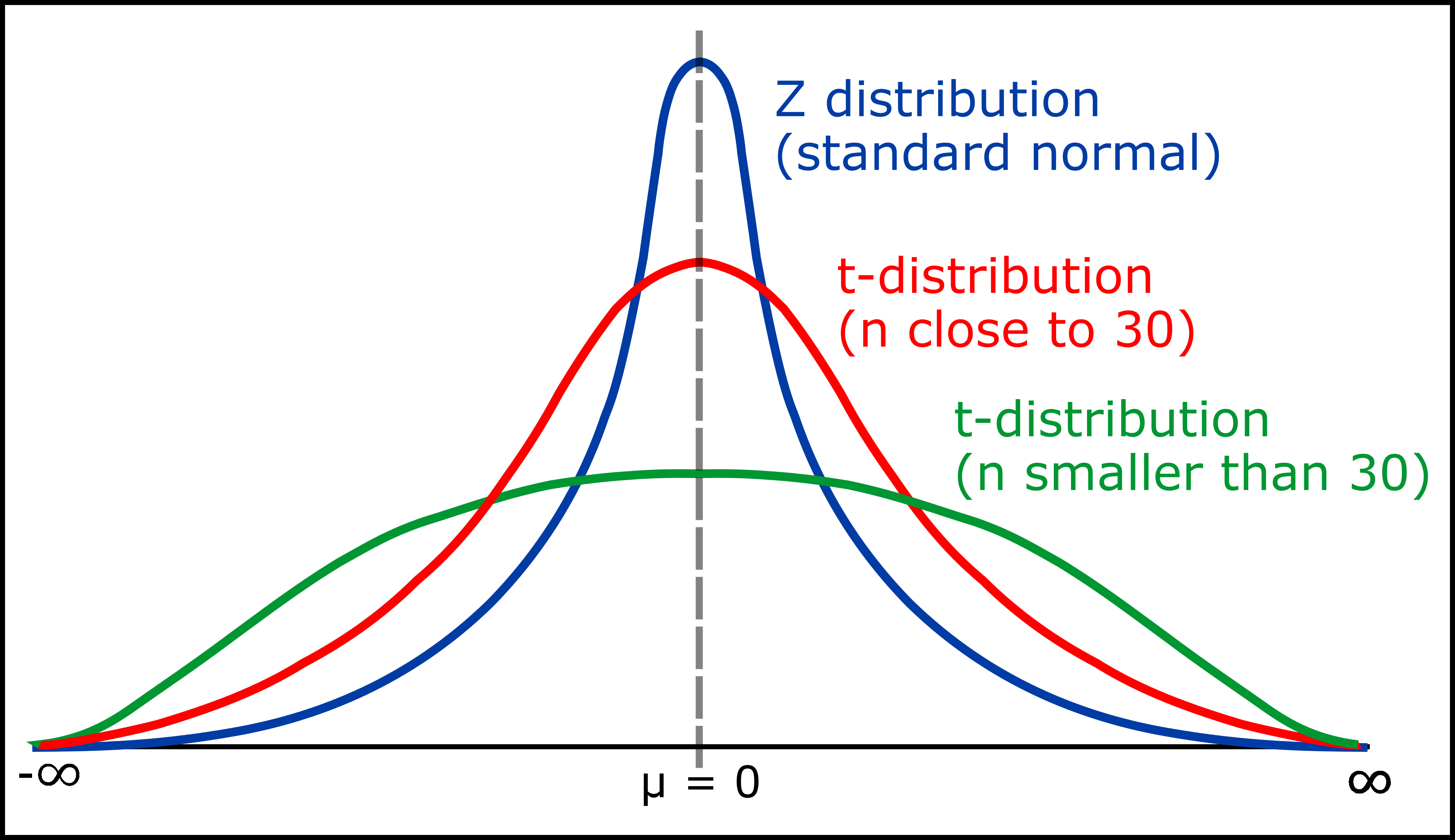# Student’s t-distribution in Statistics

• Difficulty Level : Medium
• Last Updated : 19 Jan, 2022

Student’s t-distribution or t-distribution is a probability distribution that is used to calculate population parameters when the sample size is small and when the population variance is unknown. Theoretical work on t-distribution was done by W.S. Gosset; he has published his findings under the pen name “Student“. That’s why it is called as Student’s t-test

It is the sampling distribution of the t-statistic. The values of the t-statistic is given by:

```t = [ x̄ - μ ] / [ s / sqrt( n ) ]

where,
t = t score
x̄ = sample mean,
μ = population mean,
s = standard deviation of the sample,
n = sample size ```

When to Use the t-Distribution?

Student’s t Distribution is used when

• The sample size must be 30 or less than 30.
• The population standard deviation(σ) is unknown.
• The population distribution must be unimodal and skewed.

Mathematical Derivation of t-Distribution :

The t-distribution has been derived mathematically under the assumption of normally distributed population and the formula or equation will be like this

#### f(t) = c(1+(t2/ν))(-ν+1) / 2

where,
c = Constant required to make the area under the curve equal to unity
ν = Degrees of freedom

So, this above equation indicates the probability density function(pdf) of t distribution for ν degrees of freedom.

Properties of the t-Distribution :The above diagram indicates that the blue color curve is a standard normal distribution curve or a Z distribution curve because the sample size(n) is greater than 30. And the red color curve is a t-distribution curve because the sample size(n) is close to 30. Similarly, the green color curve is also a t-distribution curve because the sample size(n) is smaller than 30.

The t-distribution has the following properties :

• The variable in t-distribution ranges from -∞ to +∞ (-∞ < t < +∞).
• t- distribution will be symmetric like normal distribution, if power of t is even in probability density function(pdf).
• For large values of ν(i.e increased sample size n); the t-distribution tends to a standard normal distribution. This implies that for different ν values, the shape of t-distribution also differs.
• The t-distribution is less peaked than normal distribution at the center and higher peaked in the tails. From the above diagram one can observe that the red and green curves are less peaked at the center but higher peaked at the tails than the blue curve.
• The value of y(peak height) attains highest at μ = 0 as one can observe the same in the above diagram.
• The mean of the distribution is equal to 0 for ν > 1 where ν = degrees of freedom, otherwise undefined.
• The median and mode of the distribution is equal to 0.
• The variance is equal to ν / ν-2 for ν > 2 and ∞ for 2 < ν ≤ 4 otherwise undefined.
• The skewness is equal to 0 for ν > 3, otherwise undefined.

Note –
Degrees of freedom refers to the number of independent observations in a set of data. When estimating a mean score or a proportion from a single sample, the number of independent observations is equal to the sample size minus one.
Hence, the distribution of the t statistic from samples of size 10 would be described by a t distribution having 10 – 1 or 9 degrees of freedom. Similarly, a t- distribution having 15 degrees of freedom would be used with a sample of size 16.

t-Distribution Table :
t-Distribution table gives t-value for a different level of significance and different degrees of freedom. The calculated t-value will be compared with the tabulated t-value. For example, if one is performing student’s t-test and for that performance, he has taken 5% level of significance and he got or calculated t-value and he has taken his tabulated t-value and if calculated t-value is higher than the tabulated t-value, in that case, it will say that there is a significant difference between the population mean and the sample means at 5% level of significance and if vice versa then, in that case, it will say that there is no significant difference between the population mean and the sample means at 5% level of significance. Here is the link to the t-Distribution table: http://www.ttable.org/

My Personal Notes arrow_drop_up